AnnouncementsWorkshop Information: July 14-16, 2011 University of British Columbia, Vancouver, Canada When delay effects are included in mathematical models one obtains differential equations with constant, time varying, distributed and/or state-dependent delays. Since related equations arise in a multitude of disciplines there is a need for good communication between these disciplines to avoid repetition of effort on similar problems and to ensure advances made in one application area propagate in a timely fashion to other fields. See details here. Contributed by Dr. Gabor Orosz.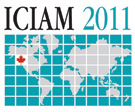Thematic Symposium on Delayed Network Dynamics July 18 – 22, 2011 Vancouver, BC, Canada An important quest of 21st-century scientists is to understand the emergent dynamics of complex physical and biological systems. Such systems are usually well studied at the component level, and the structure of the underlying networks have been described. Still, the time evolution of the systems' states is vaguely understood. One of the key elements in studying such dynamics is that finite time information propagation introduces time delays into the modeling equations. This session represents the currently arising mathematical problems through a wide range of applications in engineering and biology, including gene regulatory networks, neural networks and transportation systems. Contributed by Dr. Gabor Orosz.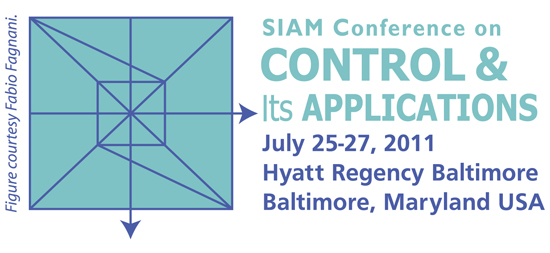http://www.siam.org/meetings/ct11/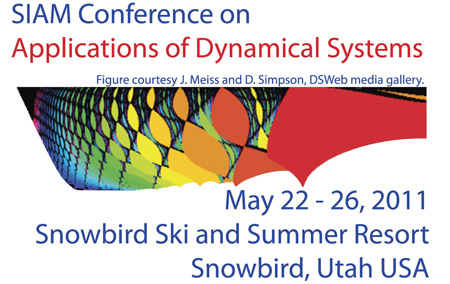http://www.siam.org/meetings/ds11/index.php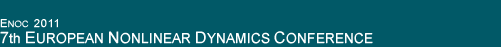http://w3.uniroma1.it/dsg/enoc2011/ Minisymposia on Systems with Time Delay Organizers: T. Kalmar Nagy (USA), K. Pyragas (Lithuania)http://www.ifac2011.org/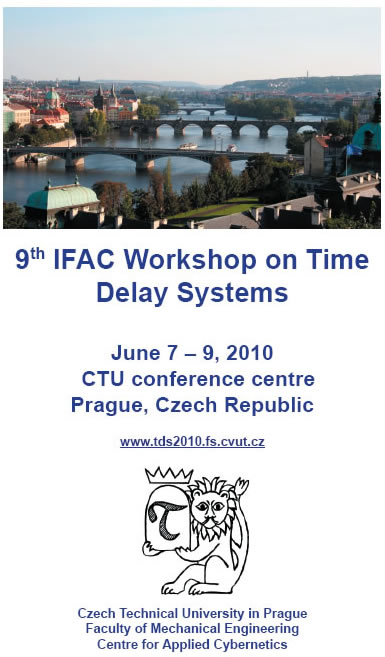www.tds2010.fs.cvut.cz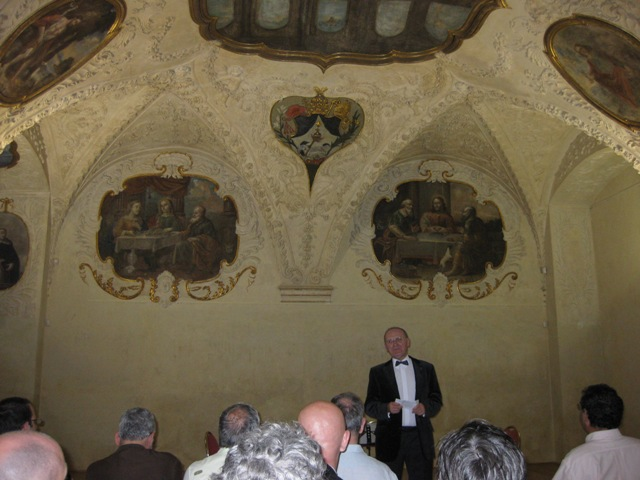Professor Pavel Zitek, the chair of the national organizing committee, is opening the banquet (June 8, 2010). (contributed by Rifat Sipahi) --------------------A snapshot from the ceiling of the Dominican monastery where the banquet took place. (contributed by Rifat Sipahi) --------------------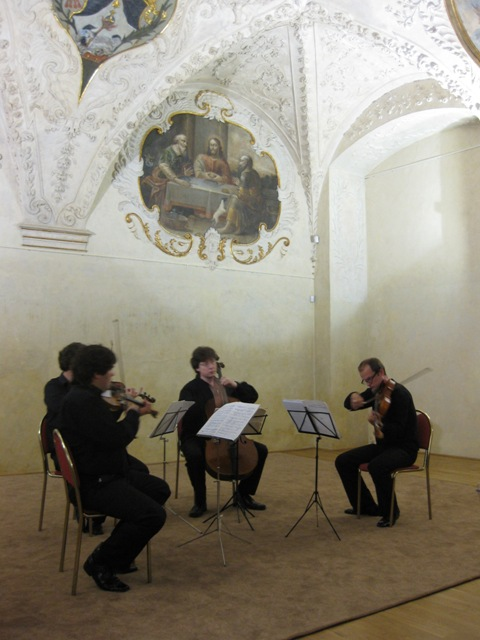A snapshot from the concert given at the Dominican monastery where the banquet took place. (contributed by Rifat Sipahi) --------------------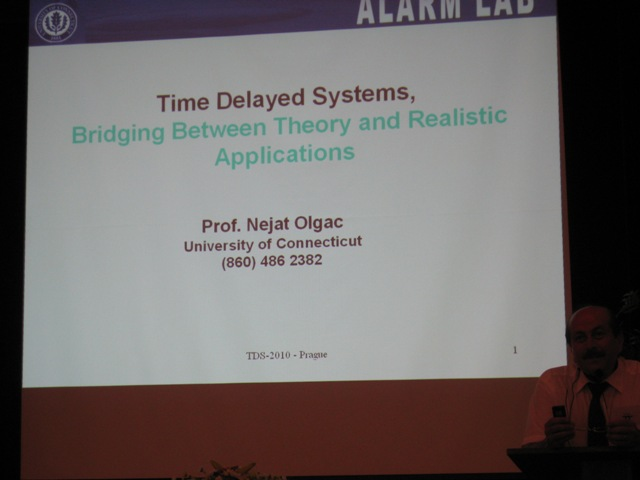Professor Nejat Olgac starting his plenary talk (contributed by Rifat Sipahi). --------------------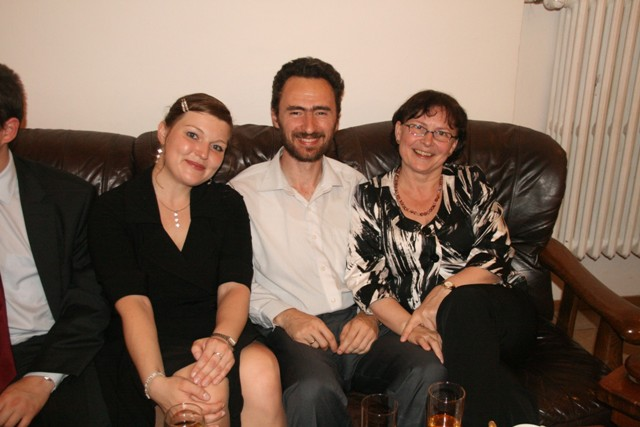Contributed by Prof. Bernhard Lampe. --------------------Contributed by Prof. Bernhard Lampe. --------------------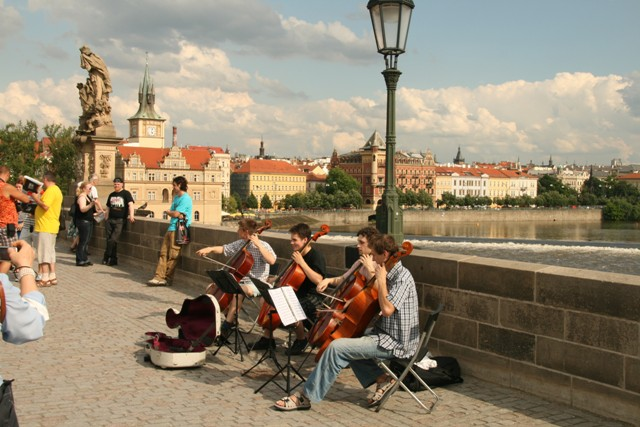A snapshot of the Charles Bridge (contributed by Prof. Bernhard Lampe). -------------------- Contributed by Miroslav Krstic Miroslav Krstic, Delay Compensation in Nonlinear, Adaptive, and PDE Systems, Birkhauser, 2009 Click to see the Table of Contents Contributed by Pierdomenico Pepe Papers presented at the 6th IFAC Workshop on Time Delay Systems, held in L'Aquila, Italy, have been published: C. Manes & P. Pepe (Eds), Proceedings of the 6th IFAC Workshop on Time-Delay Systems, L’Aquila, Italy, 2006, IFAC-PapersOnline (www.ifac-papersonline.net), Vol. 6, Part 1, ISBN 978-3-902661-11-1. Contributions Control of Delay-ODE, Delay-PDE, and PDE-ODE Cascades Miroslav Krstic Several extensions of the `predictor' (or `finite spectrum assignment') feedback are introduced, including a generalization to nonlinear ODE plants, a delay-adaptive design for LTI plants, predictor feedback for PDEs, and `predictor-like' feedback for ODEs with PDE actuator dynamics (such as reaction-diffusion or wave/string dynamics). The emphasis is on explicit construction of feedback laws and Lyapunov-Krasovskii functionals.. Click to continue (PDF) A time-delay approach for the modeling and control of plasma instabilities in thermonuclear fusion Emmanuel Witrant, Erik OLOFSSON, Silviu-Iulian NICULESCU This letter presents a summary of , where we investigated the stability problems and control issues that occur in a reversedfield pinch (RFP) device, EXTRAP-T2R, used for research in fusion plasma physics and general plasma (ionized gas) dynamics. The plant exhibits, among other things, magnetohydrodynamic instabilities known as resistive-wall modes (RWMs), growing on a time-scale set by a surrounding non-perfectly conducting shell. We propose a new modeling approach that takes into account experimental constraints, such as the actuators dynamics and control latencies leading to a multivariable time-delay model of the system. The open-loop field-error characteristics are estimated and a stability analysis of the resulting closed-loop delay differential equation (DDE) emphasizes the importance of the delay effects. We then design an optimal PID controller (its structure being constrained by the intelligent-shell (IS) control architecture) that achieves a direct eigenvalue optimization of the corresponding DDE. The presented results are substantially based on and compared with experimental data. Click to continue (PDF) Theses The spectrum of delay-differential equations: numerical methods, stability and perturbation Elias Jarlebring, T.U. Braunschweig (now at K.U. Leuven) http://www.cs.kuleuven.be/~elias Supervisor: Prof. Dr. Heike Fassbender, T.U. Braunschweig http://www.icm.tu-bs.de/~hfassben Abstract: Three types of problems related to time-delay systems are treated in this thesis. In our context, a time-delay system, or sometimes delay-differential equation (DDE), is a generalization of an ordinary differential equation (ODE) with constant coefficients. For DDEs, unlike ODEs, the derivative of the state at some time-point is not only dependent on the state at that time-point, but also on one or more previous states.    We first consider the problem of numerically computing the eigenvalues of a DDE, i.e., finding solutions of the characteristic equation, here referred to as the delay eigenvalue problem. Unlike standard ODEs, the characteristic equation of a DDE contains an exponential term. Because of this nonlinear term, the delay eigenvalue problem belongs to a class of problems referred to as nonlinear eigenvalue problems. An important contribution of the first part of this thesis is the application of a projection method for nonlinear eigenvalue problems, to the author's knowledge, previously not applied to the delay eigenvalue problem. We compare this projection method with other methods, suggested in the literature, and used in software packages. This includes methods based on discretizations of the solution operator and discretizations of the equivalent boundary value problem formulation of the DDE, i.e., the infinitesimal generator. We review discretizations based on, but not limited to, linear multi-step, Runge-Kutta and spectral collocation. The projection method is computationally superior to all of the other tested method for the presented large-scale examples. We give interpretations of the methods based on discretizations in terms of rational approximations of the exponential function or the logarithm. Some notes regarding a special case where the spectrum can be explicitly expressed are presented. The spectrum can be expressed with a formula containing a matrix version of the Lambert W function. The formula is not new. We clarify its range of applicability, and, by counter-example, show that it does not hold in general. The second part of this thesis is related to exact stability conditions of the DDE. We find exact conditions on the delays such that the DDE has a purely imaginary eigenvalue. All those combinations of the delays such that there is a purely imaginary eigenvalue (called critical delays) are parameterized. That is, we construct a mapping consisting of computable expressions, from a simple mathematical object onto the set of all subsets of the critical delays. The mapping can be expressed explicitly with trigonometric functions for scalar DDEs. We find some new formulas and verify some formulas in the literature. In general, an evaluation of the map consists of solving a quadratic eigenvalue problem of squared dimension for non-scalar problems. The constructed eigenvalue problem is large, even for DDEs of moderate size. For that reason, we show how the computational cost for one evaluation of the map can be reduced. In particular we show that the matrix-vector product corresponding to the companion linearization of the quadratic eigenvalue problem can be computed by solving a Lyapunov equation. Most of the results in the chapter on critical delays are derived for retarded DDEs as well as neutral DDEs with an arbitrary number of delays. The third and last part of this thesis is about perturbation results for nonlinear eigenvalue problems. We discuss some results in eigenvalue perturbation theory which can be generalized to nonlinear eigenvalue problems. A sensitivity formula for the movement of the eigenvalues extends nicely to nonlinear eigenvalue problems. We introduce a fixed point form for the nonlinear eigenvalue problem, and show that some methods in the literature can be interpreted as set-valued fixed point iterations. The convergence order of these types of iterations can be determined from an expression containing the left and right eigenvectors. We also use some results from fixed point theory. The famous result in perturbation theory referred to as the Bauer-Fike theorem, can be generalized to the nonlinear eigenvalue problem if we assume that the set-valued fixed point problem has a certain contraction property. The PDF can be downloaded from the permanent link: http://www.digibib.tu-bs.de/?docid=00023961 Robust control and observation of LPV Time-Delay Systems Corentin Briat (corentin.briat@gipsa-lab.inpg.fr / corentin@briat.info) Supervisors:    Olivier Sename -  olivier.sename@gipsa-lab.inpg.fr (GIPSA-Lab Automatic Control Dpt., Grenoble, France)    Jean-françois Lafay  - Jean-Francois.Lafay@irccyn.ec-nantes.fr (IRCCyN, Nantes, France) Abstract: At the difference of finite dimensional systems, the derivation of design tools from stability results is more difficult in the framework of time-delay systems due to a larger number of decision matrices involved in the LMI conditions. This thesis is based on a particular Lyapunov-Krasovskii functional which leads to non-convex conditions when used for design purposes. Several types of relaxations are discussed and a relaxation based on the  introduction of  'slack' variables is considered. This 'oriented' relaxation has the benefit of simplifying the design problem at the expense of increasing (slightly) the conservatism and the computational complexity. Another advantage of this approach is the possibility of generalization to more complex functional such as discretized functionals, leading then to an efficient reduction of conservatism. Using these results, both memory and memoryless controllers/observers are developed and, more precisely, controllers implementing inexact delay are studied. A new type of controllers referred to as 'delay-scheduled controllers' are also introduced. In such controllers, the matrix gains are scheduled by the delay-value (as done in the LPV framework) and a robust synthesis with respect to delay uncertainty is performed. The interest of such an approach is to embed an information on the delay in the controller without any necessity of storing past values of the state (as done for controller with memory). The design of such controllers is a difficult problem which relies on several techniques that need to be improved in future works. More information can be found at http://www.lag.ensieg.inpg.fr/sename/ http://www.briat.info (a preliminary version of the thesis and the slides of the defense can be downloaded there)